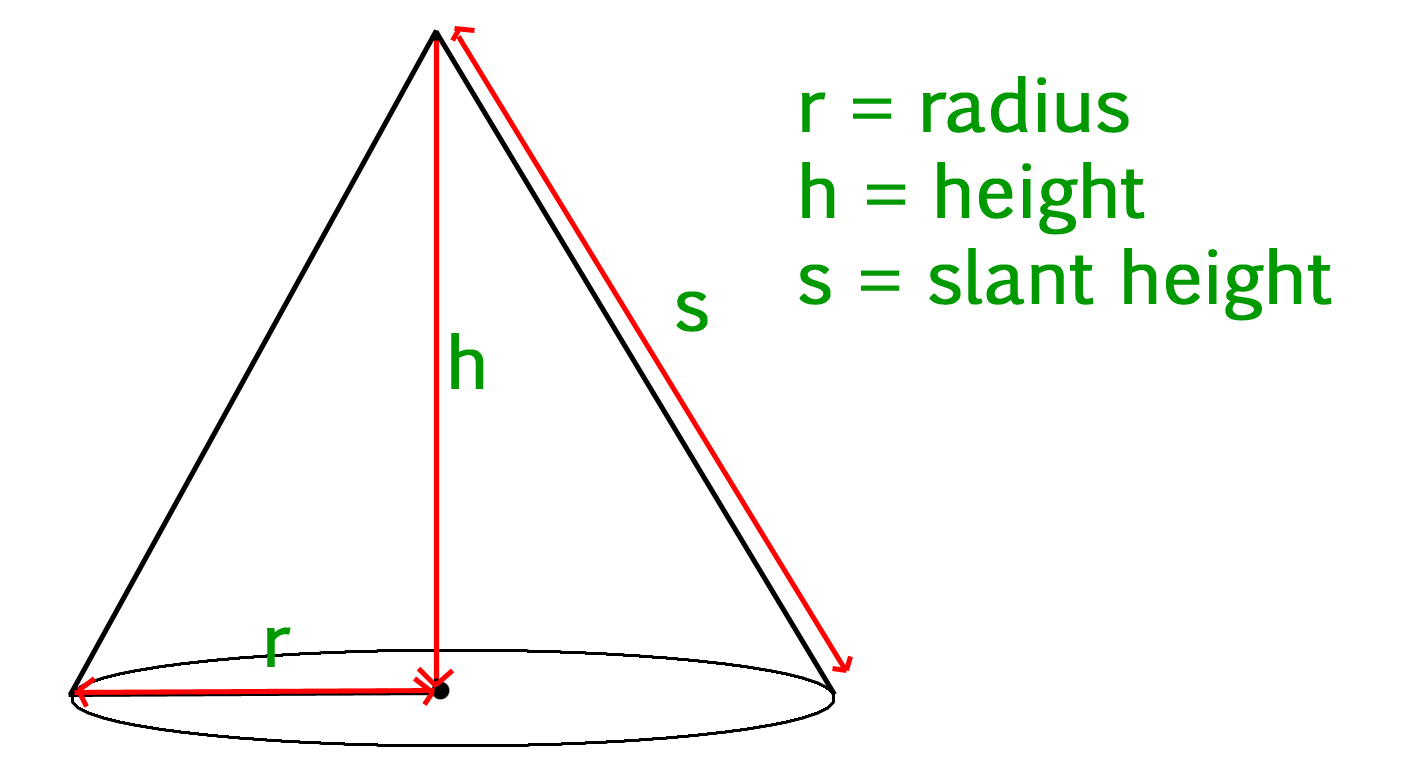# Calculate volume and surface area of a cone

Given slant height, height and radius of a cone, we have to calculate the volume and surface area of the cone.

• Cone :
Cone is a three dimensional geometric shape. It consists of a base having the shape of a circle and a curved side (the lateral surface) ending up in a tip called the apex or vertex.• Volume of a cone :
The volume of a cone is given by the formula –

`volume = 1/3(pi * r * r * h)`

where r is the radius of the circular base, and h is the height (the perpendicular distance from the base to the vertex).

• Surface area of a cone :
The surface area of a cone is given by the formula –

`area = pi * r * s + pi * r^2  `

Where r is the radius of the circular base, and s is the slant height of the cone.

• Examples :

```Input :
slant_height = 13
height = 12
Output :
Volume Of Cone = 314.159
Surface Area Of Cone = 282.743

Input :
slant_height = 10
height = 8
Output :
Volume Of Cone = 301.593
Surface Area Of Cone = 301.593
```

## C++

 `// CPP program to calculate Volume  ` `// and Surface area of Cone ` `#include ` `using` `namespace` `std; ` ` `  `float` `pi = 3.14159; ` ` `  `// Function to calculate ` `// Volume of cone ` `float` `volume(``float` `r, ``float` `h) ` `{ ` `    ``return` `(``float``(1) / ``float``(3)) * pi *  ` `                             ``r * r * h; ` `} ` ` `  `// Function to calculate ` `// Surface area of cone ` `float` `surface_area(``float` `r, ``float` `s) ` `{ ` `    ``return` `pi * r * s + pi * r * r; ` `} ` ` `  `// Driver Code ` `int` `main() ` `{ ` `    ``float` `radius = 5; ` `    ``float` `slant_height = 13; ` `    ``float` `height = 12; ` `    ``float` `vol, sur_area; ` ` `  `    ``// Printing value of volume  ` `    ``// and surface area ` `    ``cout << ``"Volume Of Cone : "` `        ``<< volume(radius, height) << endl; ` `    ``cout << ``"Surface Area Of Cone : "` `        ``<< surface_area(radius, slant_height); ` `    ``return` `0; ` `} `

## Java

 `// Java program to calculate  ` `// Volume and Surface area of cone ` `class` `GFG  ` `{ ` `    ``static` `float` `pi = ``3``.14159f; ` ` `  `    ``// Function to calculate  ` `    ``// Volume of cone ` `    ``public` `static` `float` `volume(``float` `r,  ` `                               ``float` `h) ` `    ``{ ` `        ``return` `(``float``)``1` `/ ``3` `* pi * h * ` `                               ``r * r; ` `    ``} ` ` `  `    ``// Function to calculate  ` `    ``// Surface area of cone ` `    ``public` `static` `float` `surface_area(``float` `r,  ` `                                     ``float` `s) ` `    ``{ ` `        ``return` `pi * r * s + pi * r * r; ` `    ``} ` ` `  `    ``// Driver Code ` `    ``public` `static` `void` `main(String args[]) ` `    ``{ ` `        ``float` `radius = ``5``; ` `        ``float` `slant_height = ``13``; ` `        ``float` `height = ``12``; ` `        ``float` `vol, sur_area; ` ` `  `        ``// Printing value of volume ` `        ``// and surface area ` `        ``System.out.print(``"Volume Of Cone : "``); ` `        ``System.out.println(volume(radius, height)); ` ` `  `        ``System.out.print(``"Surface Area Of Cone : "``); ` `        ``System.out.println(surface_area(radius,  ` `                                        ``slant_height)); ` `         `  `    ``} ` `} ` ` `  `// This code is contributed by "akanshgupta" `

## Python

 `''' Python3 program to calculate Volume and  ` `    ``Surface area of Cone'''` ` `  `# Importing Math library for value Of PI ` `import` `math ` `pi ``=` `math.pi ` ` `  `# Function to calculate Volume of Cone ` `def` `volume(r, h): ` `    ``return` `(``1` `/` `3``) ``*` `pi ``*` `r ``*` `r ``*` `h ` ` `  `# Function To Calculate Surface Area of Cone ` `def` `surfacearea(r, s): ` `    ``return` `pi ``*` `r ``*` `s ``+` `pi ``*` `r ``*` `r ` ` `  `# Driver Code ` `radius ``=` `float``(``5``) ` `height ``=` `float``(``12``) ` `slat_height ``=` `float``(``13``) ` `print``( ``"Volume Of Cone : "``, volume(radius, height) ) ` `print``( ``"Surface Area Of Cone : "``, surfacearea(radius, slat_height) ) `

## C#

 `// C# program to calculate  ` `// Volume and Surface area of cone ` `using` `System; ` ` `  `class` `GFG  ` `{ ` `    ``static` `float` `pi = 3.14159f; ` ` `  `    ``// Function to calculate  ` `    ``// Volume of cone ` `    ``public` `static` `float` `volume(``float` `r,  ` `                               ``float` `h) ` `    ``{ ` `        ``return` `(``float``)1 / 3 * pi * h *  ` `                               ``r * r; ` `    ``} ` ` `  `    ``// Function to calculate  ` `    ``// Surface area of cone ` `    ``public` `static` `float` `surface_area(``float` `r,  ` `                                     ``float` `s) ` `    ``{ ` `        ``return` `pi * r * s + pi * r * r; ` `    ``} ` ` `  `    ``// Driver Code ` `    ``public` `static` `void` `Main() ` `    ``{ ` `        ``float` `radius = 5; ` `        ``float` `slant_height = 13; ` `        ``float` `height = 12; ` `        ``//float vol, sur_area; ` ` `  `        ``// Printing value of volume  ` `        ``// and surface area ` `        ``Console.Write(``"Volume Of Cone : "``); ` `        ``Console.WriteLine(volume(radius,  ` `                                 ``height)); ` ` `  `        ``Console.Write(``"Surface Area Of Cone : "``); ` `        ``Console.WriteLine(surface_area(radius,  ` `                                       ``slant_height)); ` `         `  `    ``} ` `} ` ` `  `// This code is contributed by "vt_m" `

## PHP

 ` `

Output :

```Volume Of Cone : 314.159
Surface Area Of Cone : 282.743
```

My Personal Notes arrow_drop_upCheck out this Author's contributed articles.

If you like GeeksforGeeks and would like to contribute, you can also write an article using contribute.geeksforgeeks.org or mail your article to contribute@geeksforgeeks.org. See your article appearing on the GeeksforGeeks main page and help other Geeks.

Please Improve this article if you find anything incorrect by clicking on the "Improve Article" button below.

Improved By : vt_m